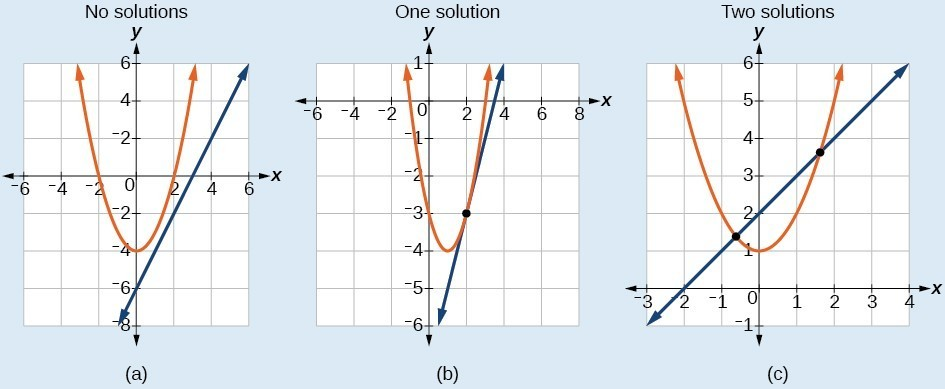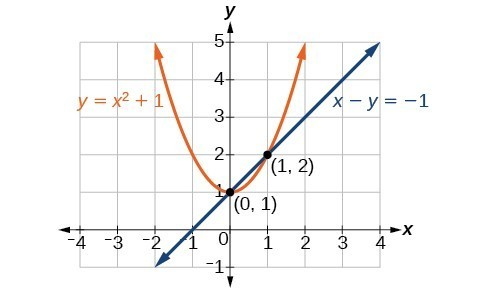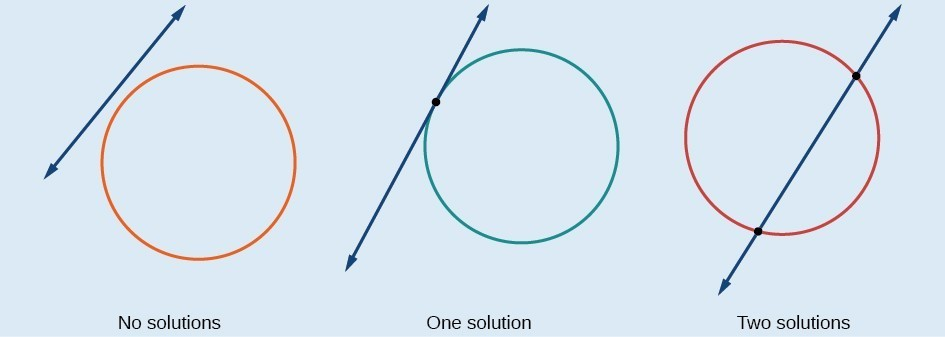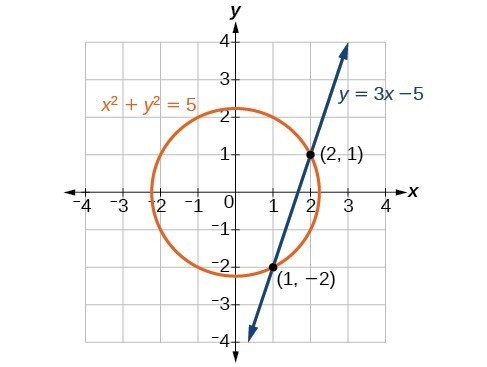## Solving a System of Nonlinear Equations Using Substitution

A system of nonlinear equations is a system of two or more equations in two or more variables containing at least one equation that is not linear. Recall that a linear equation can take the form $Ax+By+C=0$. Any equation that cannot be written in this form in nonlinear. The substitution method we used for linear systems is the same method we will use for nonlinear systems. We solve one equation for one variable and then substitute the result into the second equation to solve for another variable, and so on. There is, however, a variation in the possible outcomes.

## Intersection of a Parabola and a Line

There are three possible types of solutions for a system of nonlinear equations involving a parabola and a line.

### A General Note: Possible Types of Solutions for Points of Intersection of a Parabola and a Line

Figure 2 illustrates possible solution sets for a system of equations involving a parabola and a line.

• No solution. The line will never intersect the parabola.
• One solution. The line is tangent to the parabola and intersects the parabola at exactly one point.
• Two solutions. The line crosses on the inside of the parabola and intersects the parabola at two points.Figure 2

### How To: Given a system of equations containing a line and a parabola, find the solution.

1. Solve the linear equation for one of the variables.
2. Substitute the expression obtained in step one into the parabola equation.
3. Solve for the remaining variable.
4. Check your solutions in both equations.

### Example 1: Solving a System of Nonlinear Equations Representing a Parabola and a Line

Solve the system of equations.

$\begin{array}{l}x-y=-1\hfill \\ y={x}^{2}+1\hfill \end{array}$

### Solution

Solve the first equation for $x$ and then substitute the resulting expression into the second equation.

$\begin{array}{llll}x-y=-1\hfill & \hfill & \hfill & \hfill \\ \text{ }x=y - 1\hfill & \hfill & \hfill & \text{Solve for }x.\hfill \\ \hfill & \hfill & \hfill & \hfill \\ \text{ }y={x}^{2}+1\hfill & \hfill & \hfill & \hfill \\ \text{ }y={\left(y - 1\right)}^{2}+1\hfill & \hfill & \hfill & \text{Substitute expression for }x.\hfill \end{array}$

Expand the equation and set it equal to zero.

$\begin{array}{l}y={\left(y - 1\right)}^{2}\hfill \\ \text{ }=\left({y}^{2}-2y+1\right)+1\hfill \\ \text{ }={y}^{2}-2y+2\hfill \\ 0={y}^{2}-3y+2\hfill \\ \text{ }=\left(y - 2\right)\left(y - 1\right)\hfill \end{array}$

Solving for $y$ gives $y=2$ and $y=1$. Next, substitute each value for $y$ into the first equation to solve for $x$. Always substitute the value into the linear equation to check for extraneous solutions.

$\begin{array}{l}\text{ }x-y=-1\hfill \\ x-\left(2\right)=-1\hfill \\ \text{ }x=1\hfill \\ \hfill \\ x-\left(1\right)=-1\hfill \\ \text{ }x=0\hfill \end{array}$

The solutions are $\left(1,2\right)$ and $\left(0,1\right),\text{}$ which can be verified by substituting these $\left(x,y\right)$ values into both of the original equations.Figure 3

### Could we have substituted values for $y$ into the second equation to solve for $x$ in Example 1?

Yes, but because $x$ is squared in the second equation this could give us extraneous solutions for $x$.

For $y=1$

$\begin{array}{l}y={x}^{2}+1\hfill \\ y={x}^{2}+1\hfill \\ {x}^{2}=0\hfill \\ x=\pm \sqrt{0}=0\hfill \end{array}$

This gives us the same value as in the solution.

For $y=2$

$\begin{array}{l}y={x}^{2}+1\hfill \\ 2={x}^{2}+1\hfill \\ {x}^{2}=1\hfill \\ x=\pm \sqrt{1}=\pm 1\hfill \end{array}$

Notice that $-1$ is an extraneous solution.

### Try It 1

Solve the given system of equations by substitution.

$\begin{array}{l}3x-y=-2\hfill \\ 2{x}^{2}-y=0\hfill \end{array}$

Solution

## Intersection of a Circle and a Line

Just as with a parabola and a line, there are three possible outcomes when solving a system of equations representing a circle and a line.

### A General Note: Possible Types of Solutions for the Points of Intersection of a Circle and a Line

Figure 4 illustrates possible solution sets for a system of equations involving a circle and a line.

• No solution. The line does not intersect the circle.
• One solution. The line is tangent to the circle and intersects the circle at exactly one point.
• Two solutions. The line crosses the circle and intersects it at two points.Figure 4

### How To: Given a system of equations containing a line and a circle, find the solution.

1. Solve the linear equation for one of the variables.
2. Substitute the expression obtained in step one into the equation for the circle.
3. Solve for the remaining variable.
4. Check your solutions in both equations.

### Example 2: Finding the Intersection of a Circle and a Line by Substitution

Find the intersection of the given circle and the given line by substitution.

$\begin{array}{l}{x}^{2}+{y}^{2}=5\hfill \\ y=3x - 5\hfill \end{array}$

### Solution

One of the equations has already been solved for $y$. We will substitute $y=3x - 5$ into the equation for the circle.

$\begin{array}{c}{x}^{2}+{\left(3x - 5\right)}^{2}=5\\ {x}^{2}+9{x}^{2}-30x+25=5\\ 10{x}^{2}-30x+20=0\end{array}$

Now, we factor and solve for $x$.

$\begin{array}{l}10\left({x}^{2}-3x+2\right)=0\hfill \\ 10\left(x - 2\right)\left(x - 1\right)=0\hfill \\ x=2\hfill \\ x=1\hfill \end{array}$

Substitute the two x-values into the original linear equation to solve for $y$.

$\begin{array}{l}y=3\left(2\right)-5\hfill \\ =1\hfill \\ y=3\left(1\right)-5\hfill \\ =-2\hfill \end{array}$

The line intersects the circle at $\left(2,1\right)$ and $\left(1,-2\right)$, which can be verified by substituting these $\left(x,y\right)$ values into both of the original equations.Figure 5

### Try It 2

Solve the system of nonlinear equations.

$\begin{array}{l}{x}^{2}+{y}^{2}=10\hfill \\ x - 3y=-10\hfill \end{array}$